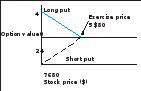### Create an Account

Already have account?

### Forgot Your Password ?

Home / Questions / The following diagram shows the value of a put option at expiration Ignoring transaction ...

# The following diagram shows the value of a put option at expiration Ignoring transaction costs, which of the following statements about the value of the put option at expiration is true

1. The following diagram shows the value of a put option at expiration:Ignoring transaction costs, which of the following statements about the value of the put option at expiration is true?

1. The expiration value of the short position in the put is \$4 if the stock price is \$76.

www.mhhe.com/bkm

2. The expiration value of the long position in the put is 2\$4 if the stock price is \$76.

3. The long put has a positive expiration value when the stock price is below \$80.

4. The value of the short position in the put is zero for stock prices equaling or exceeding \$76.

Jun 16 2020 View more View Less

#### Answer (Solved)Subscribe To Get Solution